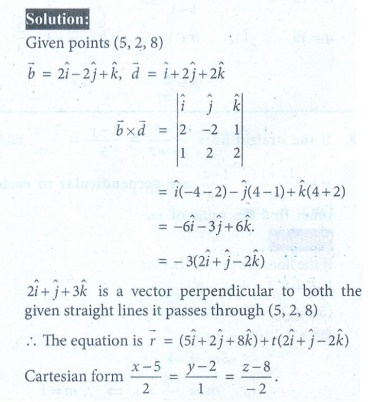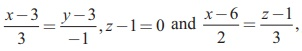Home | | Maths 12th Std | Exercise 6.5: Point of intersection of two straight lines

# Exercise 6.5: Point of intersection of two straight lines

Maths Book back answers and solution for Exercise questions - Find the parametric form of vector equation and Cartesian equations of a straight line

EXERCISE 6.5

1. Find the parametric form of vector equation and Cartesian equations of a straight line passing through (5, 2, 8) and is perpendicular to the straight lines= (iˆ + ˆj - ˆk ) + s(2iˆ - 2 ˆj + ˆk ) and= (2iˆ jˆ - 3kˆ ) + t+ 2 ˆ+ 2ˆ) .2. Show that the lines= (6iˆ + ˆj + k ) + s(iˆ + 2 ˆj - k ) and= (3ˆ+ 2 ˆ- 2ˆ) + t(2ˆ+ 4 ˆ- 5ˆ) are skew lines and hence find the shortest distance between them.3. If the two linesintersect at a point, find the value of .4. Show that the linesy-2 =0 intersect. Also find the point of intersection.5. Show that the straight lines x +1 = 2 y = -12z and x = y + 2 = 6z - 6 are skew and hence find the shortest distance between them.6. Find the parametric form of vector equation of the straight line passing through (-1, 2,1) and parallel to the straight line= (2iˆ + 3 ˆkˆ) + t(iˆ - 2 ˆkˆ) and hence find the shortest distance between the lines.7. Find the foot of the perpendicular drawn from the point (5, 4, 2) to the lineAlso, find the equation of the perpendicular.